# Sinusoidal Forcing Of A Spring-Mass-Damper System¶

This notebook introduces external forcing of a vibratory system, where the external force is modeled as a sinusoidal input to the bottom of a bus driver's seat.

After the completion of this assignment students will be able to:

• excite a system with a sinusoidal input
• understand the difference in transient and steady state solutions
• relate the frequency response to the time series
• create a frequency response plot
• define resonance and determine the parameters that cause resonance

# Introduction¶

We have only been dealing with the free response of a system given some initial coordinate (position, orientation) or speed value (velocity, angular velocity). The behavior observed is the "natural" behavior of the system, i.e. the motion that it would exhibit if no external loads act on the system. For the simple single degree of freedom systems we have dealt with so far it is possible to apply a time varying external force or torque to the system to influence the time trajectory of the single coordinate. For example let's investigate the simplest system in vibrations, a particle that can slide laterally but is fixed to a wall via a linear spring and linear damper and has a sinusoidal force applied to it as it moves:In :
import numpy as np
import matplotlib.pyplot as plt
%matplotlib inline

In :
from resonance.linear_systems import MassSpringDamperSystem

In :
sys = MassSpringDamperSystem()


The mass, $m$, stiffness of the spring, $k$, and the damping coefficient of the dashpot, $c$, can be set on this model.

In :
sys.constants

Out:
{'mass': 1.0, 'damping': 0.0, 'stiffness': 100}

# Undamped Forced Motion¶

Each system has a function called sinusoidal_forcing_response() that works in a similar fashion to free_response(). Two new pieces of information are required: the forcing amplitude, $F_o$, and the forcing frequency, $\omega$ as the first two arguments.

## Exercise¶

Set $F_o=1.0$ N and $\omega=2\pi$ rad/s and generate a trajectory for 20 seconds using sinusoidal_forcing_response(). Plot the resulting trajectories. Use sinusoidal_forcing_response? to read the documentation for the function.

In :
Fo = 1.0  # N
traj = sys.sinusoidal_forcing_response(Fo, omega, 20.0)
traj.plot(subplots=True)

Out:
array([<matplotlib.axes._subplots.AxesSubplot object at 0x7ff42b5faf40>,
<matplotlib.axes._subplots.AxesSubplot object at 0x7ff42b6e68e0>,
<matplotlib.axes._subplots.AxesSubplot object at 0x7ff42b6f2850>,
<matplotlib.axes._subplots.AxesSubplot object at 0x7ff42b6a3eb0>],
dtype=object)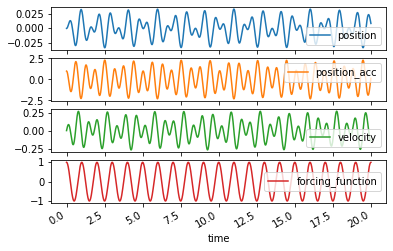In :
# write your answer here


Notice that there is no longer a simple sinusoidal oscillation. The trajactory seems that it may still be periodic but the motion is now some combination of the natural motion and the motion due to the forcing term.

## Exercise¶

Run the following cell that lets you adjust the forcing amplitude and frequency interactively. Try out a variety combinations of the two values and note what you observe with regards to the position trajectory.

In :
from ipywidgets import interact

fig, ax = plt.subplots(1, 1)
traj = sys.sinusoidal_forcing_response(0.0, 0.0, 20.0)
lines = ax.plot(traj.index, traj.position)
ax.set_xlabel('Time [s]')
ax.set_ylabel('Position [m]')
ax.set_ylim((-0.5, 0.5))

traj = sys.sinusoidal_forcing_response(amplitude, frequency, 20.0)
lines.set_data(traj.index, traj.position)

interact(adjust_forcing, amplitude=(0.0, 10.0, 0.2), frequency=(0.0, 10 * np.pi, 0.3));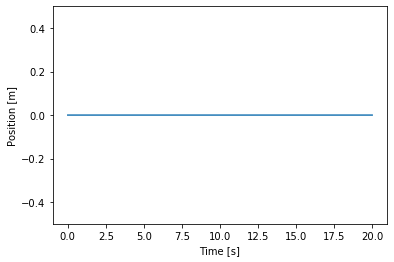# Forcing at and around the natural frequency¶

You may have notice some particularly interesting motion in a particular regime of frequencies. It turns out that if you force the system at a frequency near the natural frequency of the system. Simulate the free response of the system and find the natural frequency in radians per second. Investigate the forced trajectory with a 1 Newton forcing amplitude and a forcing frequency slightly less than, slightly more than, and exactly at the natural frequency.

In :
sys.coordinates['position'] = 0.1  # m
traj = sys.free_response(5.0)
traj.plot(subplots=True);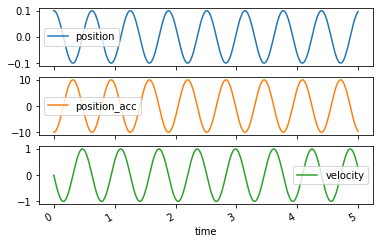## Exercise¶

Estimate the natural frequency using any method you'd like.

In :
from resonance.functions import estimate_period
2 * np.pi / estimate_period(traj.index, traj.position)

Out:
9.995976625058434
In :
m, c, k = sys.canonical_coefficients()
np.sqrt(k/m)

Out:
10.0
In :
# write your answer here


## Just lower than the natural frequency¶

Set the initial conditions to zero.

In :
sys.coordinates['position'] = 0.0  # m
sys.states

Out:
{'position': 0.0, 'velocity': 0.0}
In :
omega = 9.8  # rad/s
traj = sys.sinusoidal_forcing_response(1.0, omega, 40.0)
traj.plot(subplots=True);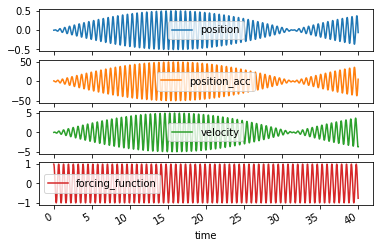## Just higher than the natural frequency¶

In :
omega = 10.2  # rad/s
traj = sys.sinusoidal_forcing_response(1.0, omega, 40.0)
traj.plot(subplots=True);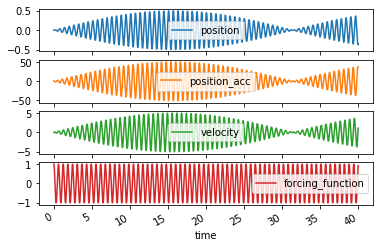This double frequency effect is called beating. Beating signals seem to have two primary frequencies, one that is much slower than the other. There is a rapid frequency that is close to the forced frequency and then another that can be estimated with:

$$\omega_{beat} = |\omega_n - \omega|$$

### Exercise¶

Calculate the beat frequency and beat period from the above equation and see if it matches the trajectory in the above plot.

In :
w_beat = np.abs(9.9959766250584341 - 10.2)

print('Beat period:', 2 * np.pi / w_beat, 's')

Beat frequency: 0.2040233749415652 rad/s
Beat period: 30.796399231113433 s

In :
# write you answer here


## Exactly at the natural frequency¶

### Exercise¶

Simulate the system with $F_o=1.0$ and $\omega=\omega_n$ for 100 seconds.

What do you observe in this case?

In :
traj = sys.sinusoidal_forcing_response(1.0, 9.9959766250584341, 100.0)
traj.plot(subplots=True);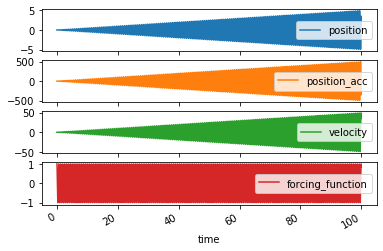In :
# write your answer here


# Forcing with Damping¶

There is also interesting vibrational behavior when both damping and forcing are introduced to the system.

### Exercise¶

Set $m=100$ kg, $c=100$ kg/s, and $k=910$ N/m and simulate the system's free response for 20 seconds to remind yourself of the behavior. Use an initial position of 0.001 m and initial velocity of 0.02 m/s. What type of vibration is this? (over-, under-, critically-damped, etc). Note that the natural frequency is:

In :
print('Natural frequency:', np.sqrt(910/100), 'rad/s')

Natural frequency: 3.0166206257996713 rad/s

In :
sys.constants['mass'] = 100  # kg
sys.constants['damping'] = 100  # kg/s
sys.constants['stiffness'] = 910  # N/m

sys.coordinates['position'] = 0.001  # m
sys.speeds['velocity'] = 0.02  # m/s

traj = sys.free_response(20)
traj.plot(subplots=True)

print("This a underdamped vibration.")

This a underdamped vibration.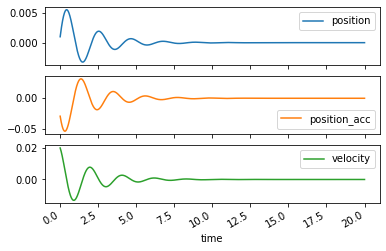In :
# write your answer here


# Forcing a damped system below it's natural frequency¶

### Exercise¶

Subject the system to $F(t) = 10 \cos(1t)$ with the same initial conditions as the previous case. This frequency is well below the natural frequency. Simulate for 20, and 50 seconds. What do you observe about the trajectories?

In :
traj = sys.sinusoidal_forcing_response(10.0, 1.0, 20)
traj.plot(subplots=True)

traj = sys.sinusoidal_forcing_response(10.0, 1.0, 50)
traj.plot(subplots=True)

Out:
array([<matplotlib.axes._subplots.AxesSubplot object at 0x7ff4296a4c70>,
<matplotlib.axes._subplots.AxesSubplot object at 0x7ff4299bd970>,
<matplotlib.axes._subplots.AxesSubplot object at 0x7ff42986c8e0>,
<matplotlib.axes._subplots.AxesSubplot object at 0x7ff42987cf40>],
dtype=object)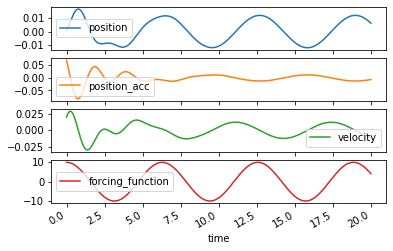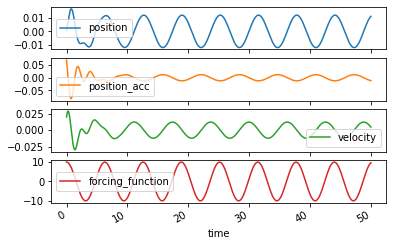In :
# write your answer here


# Just below the natural frequency with damping¶

Subject the system to $F(t) = 10 \cos(3t)$ with the same initial conditions as the previous case. This frequency is well below the natural frequency. Simulate for 20 seconds.

In :
traj = sys.sinusoidal_forcing_response(10.0, 3.0, 20)
traj.plot(subplots=True);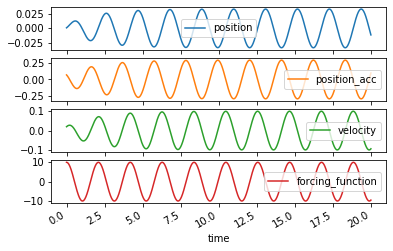# Higher than natural frequency with damping¶

Subject the system to $F(t) = 10 \cos(10t)$ with the same initial conditions as the previous case. This frequency is much higher than the natural frequency. Simulate for 20 seconds.

In :
traj = sys.sinusoidal_forcing_response(10.0, 10.0, 20)
traj.plot(subplots=True);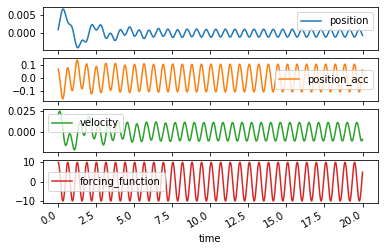### Exercise¶

What do you observe about how the vibration behavior changes with respect to the forcing amplitude and frequency? How does the amplitude and frequency of the position trajectory change with respect to the forcing function? Use the interactive plot below to adjust the forcing amplitude and frequency and observe the change in position to validate your answer.

In :
from ipywidgets import interact

fig, axes = plt.subplots(2, 1, sharex=True)
traj = sys.sinusoidal_forcing_response(0.0, 0.0, 20.0)
pos_line, = axes.plot(traj.index, traj.position)
for_line, = axes.plot(traj.index, traj.forcing_function)
axes.set_xlabel('Time [s]')
axes.set_ylabel('Position [m]')
axes.set_ylim((-0.05, 0.05))
axes.set_ylabel('Forcing [N]')
axes.set_ylim((-50, 50))

traj = sys.sinusoidal_forcing_response(amplitude, frequency, 20.0)
pos_line.set_data(traj.index, traj.position)
for_line.set_data(traj.index, traj.forcing_function)

interact(adjust_forcing, amplitude=(0.0, 50.0, 0.2), frequency=(0.0, 10, 0.1));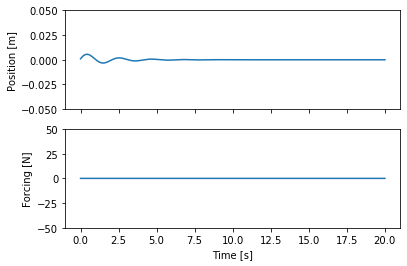In :
# write answer here


# Transient and Steady State Behavior¶

When you increase the simulation time long enough in all of the above forced responses, you should see that there is more unpredictable behavior at the beginning of the response and that in the later portion of the response the system gets into a very predictable sinusoidal motion. What is the frequency of the motion after about 10 seconds of simulation? Do you recognize this frequency?

In :
# write answer here


The underdamped regime of motion for this system occurs with a damping coefficient value as about $60.33 < c < 603.32$ kg/s.

### Exercise¶

Using the steady state behavior of the position trajectory ($10 s < t < 20$s or so, use traj[m:] to get just the last m seconds). With the damping ratio set at 100 kg/s make a plot of the position amplitude as a function of forcing frequences $1.0 < \omega < 10.0$ rad/s. Use a forcing amplitude of $F_o=10$ N. Plot a vertical line using ax.axvline(). What do you observe?

Fo = 10.0  # N

frequencies = np.linspace(1.0, 10.0, num=100)

sys.constants['damping'] = 100  # kg/s

amplitudes = []

for omega in frequencies:
traj = sys.sinusoidal_forcing_response(Fo, omega, 20.0)
amplitude = # write the code to calculate the amplitude from the trajectories here
amplitudes.append(amplitude)

fig, ax = plt.subplots(1, 1, sharex=True)
ax.set_xlabel('$\omega$ [rad/s]')

# write the plot commands for the vertical line here

In :
Fo = 10.0  # N

frequencies = np.linspace(1.0, 10.0, num=100)

sys.constants['damping'] = 100  # kg/s

amplitudes = []

for omega in frequencies:
traj = sys.sinusoidal_forcing_response(Fo, omega, 20.0)
amplitude = np.max(traj['position'][10.0:])
amplitudes.append(amplitude)

amplitudes = np.array(amplitudes)

fig, ax = plt.subplots(1, 1, sharex=True)
ax.set_xlabel('$\omega$ [rad/s]')

ax.axvline(np.sqrt(sys.constants['stiffness'] / sys.constants['mass']), color='black')
ax.plot(frequencies, amplitudes);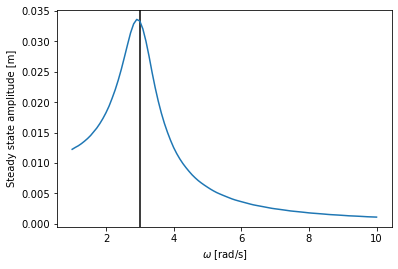In :
# write you answer here


Now let's plot a new line on the plot for different values of damping coefficients, instead of a single value. You can use a nested loop to cycle through frequencies for each damping ratio. Use 5 equally spaced values of $60 < c < 600$ and the same parameters as above. Add a legend to show which color line corresponds to what damping coefficient.

In :
Fo = 10.0  # N

dampings = np.linspace(60.0, 600.0, num=5)
frequencies = np.linspace(1.0, 10.0, num=100)
results = []

for c in dampings:

sys.constants['damping'] = c

amplitudes = []

for omega in frequencies:
traj = sys.sinusoidal_forcing_response(Fo, omega, 20.0)
amplitude = np.max(traj['position'][10.0:])
amplitudes.append(amplitude)

amplitudes = np.array(amplitudes)

results.append(amplitudes)

fig, ax = plt.subplots(1, 1, sharex=True)
ax.set_xlabel('$\omega$ [rad/s]')

ax.axvline(np.sqrt(sys.constants['stiffness'] / sys.constants['mass']), color='black')

for amps in results:
ax.plot(frequencies, amps)

ax.legend(dampings);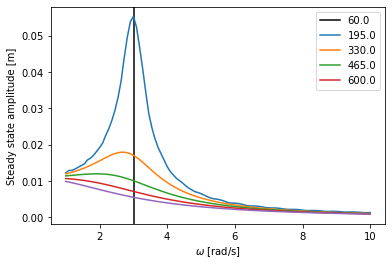In :
# write your answer here


## The frequency response¶

The plot you created above is called a frequency response plot it shows how the magnitude of the steady state response to a sinusoidal forcing. The X axis is the forcing frequency and the Y axis is the amplitude of the position oscillation. The frequency response plot also typically includes a plot of the position's steady state phase shift angle as a function of the forcing frequency too.

### Exercise¶

Read the help for frequency_plot? and create a frequency response plot for $c=60$ and $F0=10$.

sys.constants['damping'] = 60.0 Fo = 10.0 sys.frequency_response_plot(Fo);
In :
# write answer here


### Exercise¶

Create an interactive plot using the sys.frequency_response() function with a slider for the damping coefficient that goes from $60 < c < 600$ with an appropriate interval spacing. Explore how damping affects the

In :
sys.constants['damping'] = 60
axes = sys.frequency_response_plot(10.0)
amp_line = axes.lines
phase_line = axes.lines
frequencies = amp_line.get_xdata()

def update(zeta):
wn = np.sqrt(sys.constants['stiffness']/sys.constants['mass'])
c = zeta*2*sys.constants['mass']*wn
sys.constants['damping'] = c
amp, phase = sys.frequency_response(frequencies, 10.0)
amp_line.set_ydata(amp)

<function __main__.update(zeta)>